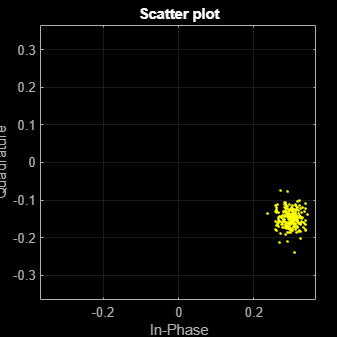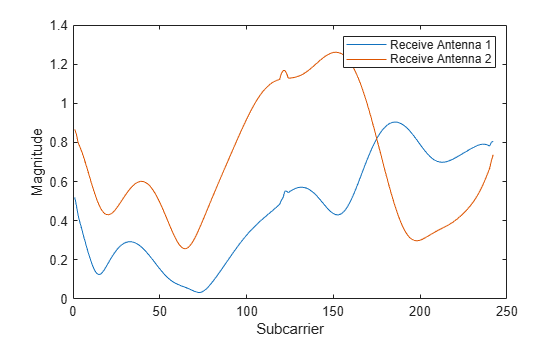# wlanVHTLTFChannelEstimate

Channel estimation using VHT-LTF

## Syntax

``chEst = wlanVHTLTFChannelEstimate(demodSig,cfg)``
``chEst = wlanVHTLTFChannelEstimate(demodSig,cbw,numSTS)``
``chEst = wlanVHTLTFChannelEstimate(___,span)``

## Description

example

````chEst = wlanVHTLTFChannelEstimate(demodSig,cfg)` returns the channel estimate, using the demodulated VHT-LTF signal, `demodSig`, given the parameters specified in `wlanVHTConfig` object `cfg`.```

example

````chEst = wlanVHTLTFChannelEstimate(demodSig,cbw,numSTS)` returns the channel estimate for the specified channel bandwidth, `cbw`, and the number of space-time streams, `numSTS`.```

example

````chEst = wlanVHTLTFChannelEstimate(___,span)` specifies the span of a moving-average filter used to perform frequency smoothing.```

## Examples

collapse all

Display the channel estimate of the data and pilot subcarriers for a VHT format channel using its long training field.

Create a VHT format configuration object. Generate a VHT-LTF based on `cfg`.

```cfg = wlanVHTConfig; txSig = wlanVHTLTF(cfg);```

Multiply the transmitted VHT-LTF signal by 0.3 - 0.15i and pass it through an AWGN channel having a 30 dB signal-to-noise ratio. Demodulate the received signal.

```rxSig = awgn(txSig*(0.3-0.15i),30); demodSig = wlanVHTLTFDemodulate(rxSig,cfg);```

Estimate the channel response using the demodulated VHT-LTF signal.

`est = wlanVHTLTFChannelEstimate(demodSig,cfg);`

Plot the channel estimate.

```scatterplot(est) grid```The channel estimate matches the complex channel multiplier.

Estimate and display the channel coefficients of a 4x2 MIMO channel using the VHT-LTF.

Create a VHT format configuration object for a channel having four spatial streams and four transmit antennas. Transmit a complete VHT waveform.

```cfg = wlanVHTConfig('NumTransmitAntennas',4, ... 'NumSpaceTimeStreams',4,'MCS',5); txWaveform = wlanWaveformGenerator([1;0;0;1;1;0],cfg);```

Set the sampling rate, and then pass the transmitted waveform through a 4x2 TGac channel.

```fs = 80e6; tgacChan = wlanTGacChannel('SampleRate',fs, ... 'NumTransmitAntennas',4,'NumReceiveAntennas',2); rxWaveform = tgacChan(txWaveform);```

Determine the VHT-LTF field indices and demodulate the VHT-LTF from the received waveform.

```indVHTLTF = wlanFieldIndices(cfg,'VHT-LTF'); ltfDemodSig = wlanVHTLTFDemodulate(rxWaveform(indVHTLTF(1):indVHTLTF(2),:), cfg);```

Generate the channel estimate by using the demodulated VHT-LTF signal. Specify a smoothing filter span of five subcarriers.

`est = wlanVHTLTFChannelEstimate(ltfDemodSig,cfg,5);`

Plot the magnitude response of the first space-time stream for both receive antennas. Due to the random nature of the fading channel, your results may vary.

```plot(abs(est(:,1,1))) hold on plot(abs(est(:,1,2))) xlabel('Subcarrier') ylabel('Magnitude') legend('Rx Antenna 1','Rx Antenna 2')```Recover bits from the VHT-Data field of a VHT multi-user transmission recovered from a fading MU-MIMO channel by using channel estimation on the VHT-LTF.

This example can return high bit error rates because the transmission does not include precoding to mitigate the interference between space-time streams. However, the example shows a typical VHT signal recovery workflow and appropriate syntax use for the functions involved.

Configure a VHT transmission with a channel bandwidth of 160 MHz, two users, and four transmit antennas. Assign one space-time stream to the first user and three space-time streams to the second user.

```cbw = 'CBW160'; numSTS = [1 3]; cfgVHT = wlanVHTConfig('ChannelBandwidth',cbw,'NumUsers',2, ... 'NumTransmitAntennas',4,'NumSpaceTimeStreams',numSTS);```

Generate a payload of bits for each user. This payload must be in the a 1-by-N cell array, where N is the number of users.

```psduLength = 8*cfgVHT.PSDULength; numUsers = cfgVHT.NumUsers; bits = cell(1,2); for nu = 1:numUsers bits{nu} = randi([0 1],psduLength(nu),1); end```

Generate VHT-LTF and VHT-Data field signals.

```txLTF = wlanVHTLTF(cfgVHT); txDataSym = wlanVHTData(bits,cfgVHT);```

Pass the VHT-Data field signal for the first user through a 4x1 channel because this signal consists of a single space-time stream. Pass the VHT-Data field for the second user data through a 4x3 channel because this signal consists of three space-time streams. Apply AWGN to each signal, assuming an SNR of 15 dB.

```snr = 15; H{1} = complex(randn(4,1),randn(4,1))/sqrt(2); H{2} = complex(randn(4,3),randn(4,3))/sqrt(2); number = zeros(2,1); ratio = zeros(2,1); for userIdx = 1:numUsers rxDataSym = awgn(txDataSym*H{userIdx},snr,'measured');```

Apply the same channel processing to the VHT-LTF for each user.

` rxLTF = awgn(txLTF*H{userIdx},snr,'measured');`

Calculate the received signal power for each user and estimate the noise variance.

``` powerDB = 10*log10(var(rxDataSym)); noiseVarEst = mean(10.^(0.1*(powerDB-snr)));```

Estimate the channel characteristics by using the VHT-LTF.

``` demod = wlanVHTLTFDemodulate(rxLTF,cbw,numSTS); chEst = wlanVHTLTFChannelEstimate(demod,cbw,numSTS);```

Recover the bits from the received VHT-Data field for each user and determine the bit error rate by comparing the recovered bits with the original payload bits.

``` dataBits = wlanVHTDataRecover(rxDataSym,chEst,noiseVarEst,cfgVHT,userIdx); [number(userIdx),ratio(userIdx)] = biterr(bits{userIdx},dataBits); disp(number(userIdx)) disp(ratio(userIdx)) end```
``` 4269 ```
``` 0.5082 ```
``` 2444 ```
``` 0.0968 ```

## Input Arguments

collapse all

Demodulated VHT-LTF signal, specified as an NST-by-NSYM-by-NR array. NST is the number of occupied subcarriers, NSYM is the number of VHT-LTF OFDM symbols, and NR is the number of receive antennas.

Data Types: `double`
Complex Number Support: Yes

Format configuration, specified as a `wlanVHTConfig` object.

Channel bandwidth, specified as `'CBW20'`, `'CBW40'`, `'CBW80'`, or `'CBW160'`. If the transmission has multiple users, the same channel bandwidth is applied to all users.

Data Types: `char` | `string`

Number of space-time streams in the transmission, specified as a scalar or vector.

• For a single user, the number of space-time streams is a scalar integer from 1 to 8.

• For multiple users, the number of space-time streams is a 1-by-NUsers vector of integers from 1 to 4, where the vector length, NUsers, is an integer from 1 to 4.

Example: `[1 3 2]` indicates that one space-time stream is assigned to user 1, three space-time streams are assigned to user 2, and two space-time streams are assigned to user 3.

Note

The sum of the space-time stream vector elements must not exceed eight.

Data Types: `double`

Filter span of the frequency smoothing filter, specified as an odd integer. The span is expressed as a number of subcarriers.

Note

If adjacent subcarriers are highly correlated, frequency smoothing results in significant noise reduction. However, in a highly frequency-selective channel, smoothing can degrade the quality of the channel estimate.

Data Types: `double`

## Output Arguments

collapse all

Channel estimate between all combinations of space-time streams and receive antennas, returned as an NST-by-NSTS,total-by-NR array. NST is the number of occupied subcarriers. NSTS,total is the total number of space-time streams for all users. For the single-user case, NSTS,total = NSTS. NR is the number of receive antennas. The channel estimate includes coefficients for both the data and pilot subcarriers.

Data Types: `double`
Complex Number Support: Yes

collapse all

### VHT-LTF

The very high throughput long training field (VHT-LTF) is located between the VHT-STF and VHT-SIG-B portion of the VHT packet.It is used for MIMO channel estimation and pilot subcarrier tracking. The VHT-LTF includes one VHT long training symbol for each spatial stream indicated by the selected MCS. Each symbol is 4 μs long. A maximum of eight symbols are permitted in the VHT-LTF.

For a detailed description of the VHT-LTF, see section 21.3.8.3.5 of IEEE® Std 802.11™-2016.

 IEEE Std 802.11ac™-2013 IEEE Standard for Information technology — Telecommunications and information exchange between systems — Local and metropolitan area networks — Specific requirements — Part 11: Wireless LAN Medium Access Control (MAC) and Physical Layer (PHY) Specifications — Amendment 4: Enhancements for Very High Throughput for Operation in Bands below 6 GHz.

 IEEE Std 802.11™-2012 IEEE Standard for Information technology — Telecommunications and information exchange between systems — Local and metropolitan area networks — Specific requirements — Part 11: Wireless LAN Medium Access Control (MAC) and Physical Layer (PHY) Specifications.

 Perahia, E., and R. Stacey. Next Generation Wireless LANs: 802.11n and 802.11ac. 2nd Edition, United Kingdom: Cambridge University Press, 2013.

## Support평가판 신청